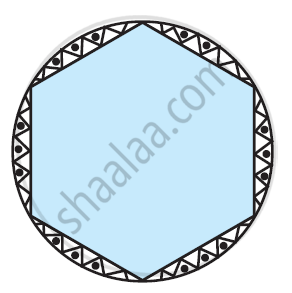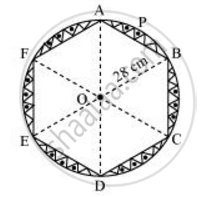Share

# A round table cover has six equal designs as shown in figure. If the radius of the cover is 28 cm, find the cost of making the designs at the rate of Rs.0.35 per cm^2. - CBSE Class 10 - Mathematics

ConceptAreas of Sector and Segment of a Circle

#### Question

A round table cover has six equal designs as shown in figure. If the radius of the cover is 28 cm, find the cost of making the designs at the rate of Rs.0.35 per cm2. [Use sqrt3 = 1.7]#### SolutionIt can be observed that these designs are segments of the circle.

Consider segment APB. Chord AB is a side of the hexagon. Each chord will substitute 360º/6 = 60º at the centre of the circle.

In ΔOAB,

∠OAB = ∠OBA (As OA = OB)

∠AOB = 60°

∠OAB + ∠OBA + ∠AOB = 180°

2∠OAB = 180° − 60° = 120°

∠OAB = 60°

Therefore, ΔOAB is an equilateral triangle.

Area of ΔOAB = sqrt3/4 xx ("side")^2

=sqrt3/4 xx (28)^2 = 196sqrt3 = 196 xx 1.7 = 333.2 cm^2

Area of sector OAPB = 60^@/360^@ xx pir^2

= 1/6xx 22/7xx28xx28

= 1232/3 cm^2

Area of segment APB = Area of sector OAPB − Area of ΔOAB

=(1232/3 - 333.2) cm^2

Therefore are of designs = 6xx(1232/3 - 333.2) cm^2

= (2464 - 1999.2)cm^2

= 464.8 cm2

Cost of making 1 cm2 designs = Rs 0.35

Cost of making 464.76 cm2 designs = 464.8 x  0.35 = Rs 162.68

Therefore, the cost of making such designs is Rs 162.68.

Is there an error in this question or solution?

#### APPEARS IN

NCERT Solution for Mathematics Textbook for Class 10 (2019 to Current)
Chapter 12: Areas Related to Circles
Ex. 12.20 | Q: 13 | Page no. 231
Solution A round table cover has six equal designs as shown in figure. If the radius of the cover is 28 cm, find the cost of making the designs at the rate of Rs.0.35 per cm^2. Concept: Areas of Sector and Segment of a Circle.
S